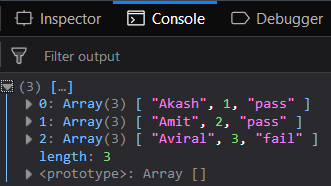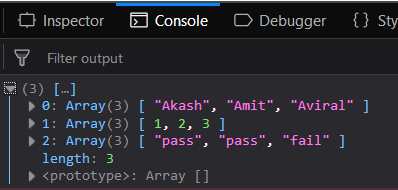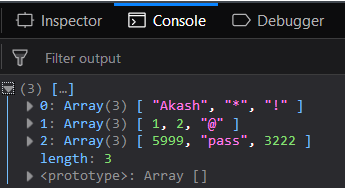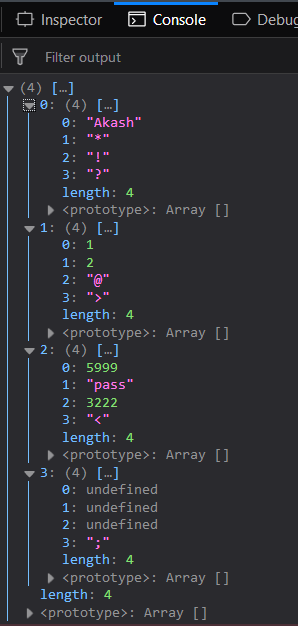Open In App
Related Articles

# Underscore.js _.zip() Function

The Underscore.js is a JavaScript library that provides a lot of useful functions like the map, filter, invoke etc even without using any built-in objects.
The _.zip() function matches each passed array of elements to the next passed array element. It is used when more than one homogeneous arrays (one type of elements like only numbers, works, special characters etc) to connect all the array by matching each element to it’s corresponding element in the next array. It can have a number of arrays as it’s parameters. The result will contain the same number of arrays as the number of elements in the largest array.

Syntax:

`_.zip( *arrays )`

Parameters: This function accepts single parameter arrays which contains array elements.

Return value: It returns the resultant arrays.

Passing homogeneous arrays to _.zip() function: The ._zip() function accepts the element from the first array and makes the first elements of the resultant array. Then, it takes the elements from the second array and maps it as the second property of that first element. This is done till all the array’s first elements are covered. Then it forms the second element of the resultant array. It runs until all the elements are covered.

Example:

 ```<``html``>``    ``<``head``>``        ``<``script` `src` `= ``"https://cdnjs.cloudflare.com/ajax/libs/underscore.js/1.9.1/underscore-min.js"` `>``        ````    ````    ``<``body``>``        ``<``script` `type``=``"text/javascript"``>``            ``console.log(_.zip(['Akash', 'Amit', 'Aviral'], ``                              ``[01, 02, 03], ``                              ``['pass', 'pass', 'fail'])``            ``);``        ````    ````                    `

Output:Passing a heterogeneous array to the _.zip() function: Pass a heterogeneous array, i.e., which contains all kinds of elements in a single array. The _.zip() function will work the same way. The procedure is the same but the output will be different. It will now form homogeneous arrays in the result.

Example:

 ```<``html``>``    ``<``head``>``        ``<``script` `src` `= ``"https://cdnjs.cloudflare.com/ajax/libs/underscore.js/1.9.1/underscore-min.js"` `>``        ````    ````    ``<``body``>``        ``<``script` `type``=``"text/javascript"``>``            ``console.log(_.zip(['Akash', 02, 'pass'], ``                              ``['Amit', 02, 'pass'], ``                              ``['Aviral', 03, 'fail'])``            ``);``        ````    ````                    `

Output:Mapping different kind of elements in _.zip() function: Passing the different kind of elements to the _.zip function. This time the process will be the same but the result will not be a homogeneous array rather it will be a heterogeneous array.

Example:

 ```<``html``>``    ``<``head``>``        ``<``script` `src` `= ``"https://cdnjs.cloudflare.com/ajax/libs/underscore.js/1.9.1/underscore-min.js"` `>``        ````    ````    ``<``body``>``        ``<``script` `type``=``"text/javascript"``>``            ``console.log(_.zip(['Akash', 01, 5999], ``                              ``['*', 02, 'pass'], ``                              ``['!', '@', 3222])``            ``);``        ````    ````                    `

Output:Passing unequal size of arrays to the _.zip() function: Passing unequal size of arrays but this will cause undefined values in the resultant arrays. This is because the number of elements who will find their matches in the rest of the arrays will be considered as a single array but if they do not found any match then they will have ‘undefined’ in place. But this will work smoothly and not give any error.

Example:

 ```<``html``>``    ``<``head``>``        ``<``script` `src` `= ``"https://cdnjs.cloudflare.com/ajax/libs/underscore.js/1.9.1/underscore-min.js"` `>``        ````    ````    ``<``body``>``        ``<``script` `type``=``"text/javascript"``>``            ``console.log(_.zip(['Akash', 01, 5999],``                              ``['*', 02, 'pass'],``                              ``['!', '@', 3222],``                              ``['?', '>', '<', ';'])``            ``);``        ````    ````                    `

Output:Note: These commands will not work in Google console or in Firefox as for these additional files need to be added which they didn’t have added. So, add the given links to your HTML file and then run them.

 `<``script` `type``=``"text/javascript"` `src` `= ``"https://cdnjs.cloudflare.com/ajax/libs/underscore.js/1.9.1/underscore-min.js"``> `` `